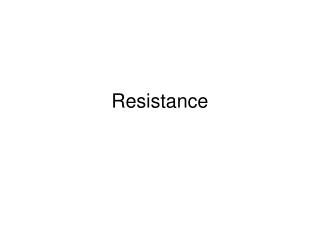DownloadDownload PresentationResistance

# Resistance

Télécharger la présentation## Resistance

- - - - - - - - - - - - - - - - - - - - - - - - - - - E N D - - - - - - - - - - - - - - - - - - - - - - - - - - -
##### Presentation Transcript

1. Resistance

2. Review of Resistors • The resistance is an intrinsic property of a material which impedes the flow of charge requiring a pd to be applied so that there can be current flow.

3. Review of Resistors • The resistance is an intrinsic property of a material which impedes the flow of charge requiring a pd to be applied so that there can be current flow. • From ohm’s law, the resistance of a device is the ratio of the potential difference across it to the current flowing through it.

4. The unit of the resistor is the ohm ( ).

5. RC Circuits

6. RC Circuits • The current in the previous circuits are time independent once the emf of the source is time independent.

7. RC Circuits • The current in the previous circuits are time independent once the emf of the source is time independent. • However we may have circuits which are time dependent. • An example is an RC circuit.

8. A RC circuit consists of a resistor R connected in series with a capacitor C.

9. The following circuit can be use the test the charging and discharging of the capacitor through the resistor.

10. Consider charging:

11. Consider charging: • Initially the capacitor is uncharged.

12. Consider charging: • Initially the capacitor is uncharged. • When in the charging position current flows and the capacitor charges. • From Kirchoff’s law:

13. Which can be written as:

14. Which can be written as: • Since • We can rewrite the equation as,

15. Which can be written as: • Since • We can rewrite the equation as, • Doing some algebra,

16. Which can be written as: • Since • We can rewrite the equation as, • Doing some algebra, • We must separate the variables so that we can integrate and find the final charge on the capacitor.

17. Separating variables,

18. Separating variables, • Integrating,

19. Separating variables, • Integrating,

20. Separating variables, • Integrating, • Which gives,

21. Taking the antilog and simplifying we get,

22. q(t) VbatC t • Taking the antilog and simplifying we get,

23. The product RC in the previous equation is called the time constant. • Has units of time. • Time taken for the charge to increase from zero to 63% of its final value.

24. Vc Vbat t • The pd across the capacitor • Which gives

25. The current for the charging • Which gives I(t) Vbat/R t

26. Consider discharging:

27. Consider discharging: • For the discharge position, the battery is no longer in the circuit.

28. Since • We can write that

29. Since • We can write that • Separating variables,

30. Since • We can write that • Separating variables, • Which in separated form is,

31. Integrating,

32. Integrating, • We get • Which after simplification is,

33. This can be written as, , noting that the initial charge is CVbat.

34. This can be written as, , noting that the initial charge is CVbat. • Differentiating gives the current, • The voltage across the capacitor is,

35. Limiting conditions: • At t=0, q= CVbat. • At t=inf, q= 0. q CVbat t

36. t I(t) Vbat t

37. Power, Energy

38. Power • The net rate of energy transfer from the source (battery) P is given by, • Power is in watts(W) or joules/second • The rate at which energy is dissipated through through the resistor is, • The energy lost is in the form of thermal energy. • The power supplied to the capacitor is,

39. Energy • The total energy supplied by the battery in a time t is given by, • The total energy dissipated in a time t, • The total energy supplied to the capacitor in time t,

40. Energy • From the conservation of energy,

41. Resistance in Series and Parallel

42. Series:

43. From the conservation of energy,

44. From the conservation of energy, • where,

45. From the conservation of energy, • where,

46. From the conservation of energy, • where,

47. In general,

48. Parallel:

49. From the conservation of charge,